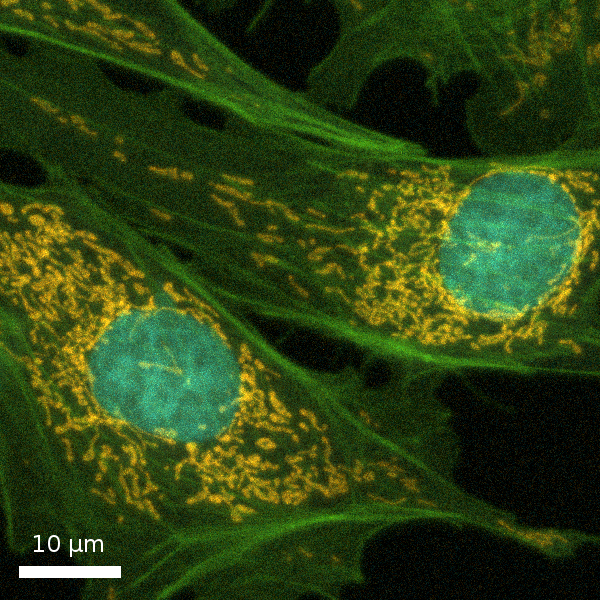Loading...

# Deconvolution

The definition of deconvolution, as used in image restoration, is that it reverses the degradative process of microscopy imaging, also known as a convolution. Deconvolution recovers the microscopy image by means of a mathematical operation. Huygens deconvolution can be applied to images from nearly every microscope type and uses the most advanced algorithms that currently exist.

The image formation process is mathematically described by a convolution equation of the form, where the image g arises from the convolution of the real light sources f (the object) and the point spread function (PSF) h. In other words, the microscope yields an image g, which is a degraded version of the object "f". The degradation is caused by blurring (convolution) by the PSF, wider PSFs leading to more severe blurring. The PSF can be measured, for example by Recording Beads, or by a theoretical optical calculation based on knowledge of the Microscopic Parameters. This is outlined in the illustration below.The aim of the deconvolution is to recover the original object "f", and we do it by doing deconvolution with a precisly defined PSF that is either accurately calculated from the image parameters or distilled from bead images using Huygens PSF Distiller.This approach is very different from blind deconvolution approach, where both the object and PSF and considered as unknown parameters

For more details on how the Huygens Software does deconvolution see Huygens Deconvolution.Raw Confocal
Huygens Deconvolved

## Testing Huygens Deconvolution Software

Are you interested in testing the latest version of the Huygens software with all its available options including deconvolution for all types microscope? Do not hesitate to request a test license.